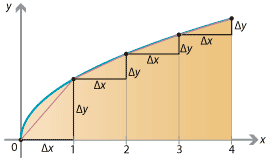### Arc length

How long is a piece of string? If the piece of string is given as a graph $$y = f(x)$$ then its length can be calculated using integration.

As we have now seen many times, we consider the curve between $$x=a$$ and $$x=b$$ and split the $$x$$-interval into $$n$$ subintervals $$[x_0, x_1]$$, $$\dots$$, $$[x_{n-1}, x_n]$$ of length $$\Delta x$$. Over each subinterval there is an arc segment that travels $$\Delta x$$ to the right and $$\Delta y$$ upwards, where $$\Delta y = f(x_j) - f(x_{j-1})$$. Approximating the arc by straight line segments, the length of each line segment is $$\sqrt{(\Delta x)^2 + (\Delta y)^2}$$. This gives an estimate for the length of the curve as

$\sum_{j=1}^n \sqrt{(\Delta x)^2 + (\Delta y)^2} = \sum_{j=1}^n \sqrt{1 + \Big( \frac{\Delta y}{\Delta x} \Big)^2} \; \Delta x.$Detailed description of diagram

Taking the limit as $$n \to \infty$$, the length of the curve is given by

$\int_a^b \sqrt{ 1 + \Big( \frac{dy}{dx} \Big)^2 } \; dx = \int_a^b \sqrt{ 1 + f'(x)^2 } \; dx.$

Next page - Links forward - Difficulties with antiderivatives